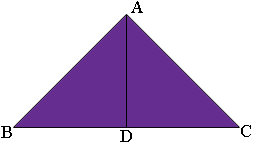SEARCH HOMEMath Central Quandaries & QueriesQuestion from imraan, a student: how to find the area of isosceles triangle by knowing only its sidesimraan,

Suppose the triangle is ABC with AB and AC the congruent sides. Let D be the midpoint of BC.What do you know about triangles ABD and ADC?

PennyMath Central is supported by the University of Regina and The Pacific Institute for the Mathematical Sciences.